# # 在 JS 中进行调试基础与示例

## # 如何进行调试

• `Resume`: 执行到下一个断点
• `Step Over`: 执行下一语句
• `Step Into`: 执行下一语句，如遇到函数，则进入函数执行
• `Step Out`: 跳出当前函数

## # Return Value

### # 如何通过调试获取函数的返回值

``````const expansiveCompute = (a, b) => a + b

function sum (a, b) {
// 在调试中如何得到 7 这个结果
return expansiveCompute(a, b)
}

sum(3, 4)
``````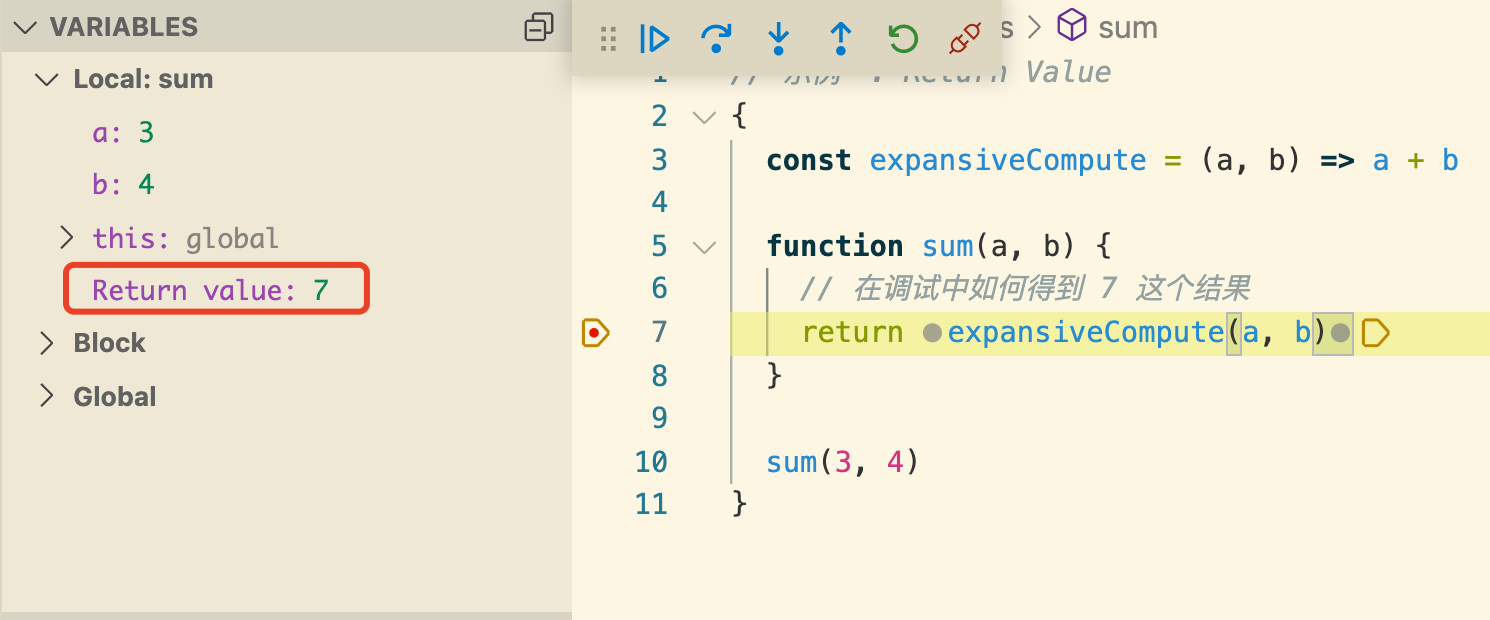## # Step Over

### # 当单行调用多个函数表达式时，`Step Over` 是跳过一行还是一个表达式？

``````// 当此行有断点时，Step Over 进入哪里
const l = [1, 2, 3, 4, 5].map(x => sum(x, 1)).filter(x => x > 3)

const n = sum(sum(3, 4), 1)
``````

## # 多层嵌套与行内断点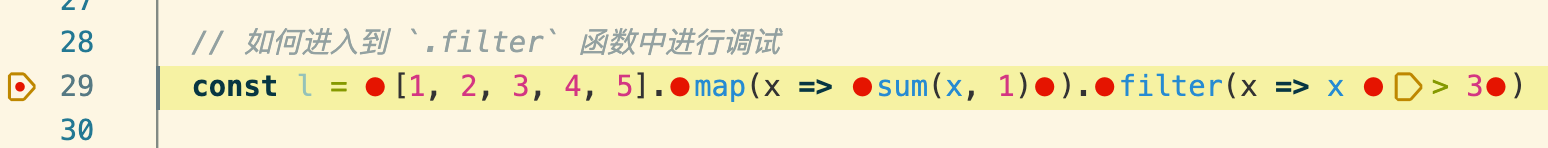### # 当单行调用多个函数表达式时，如何进入特定函数中进行 debug

(不通过对指定函数起始位置打断点的方法，因为有时无法得知指定函数位置)

``````// 如何进入到 `.filter` 函数中进行调试
const l = [1, 2, 3, 4, 5].map(x => sum(x, 1)).filter(x => x > 3)

// 如何进入到 sub 函数中进行调试
const n = sub(sum(2, sum(3, 4)), 1)
``````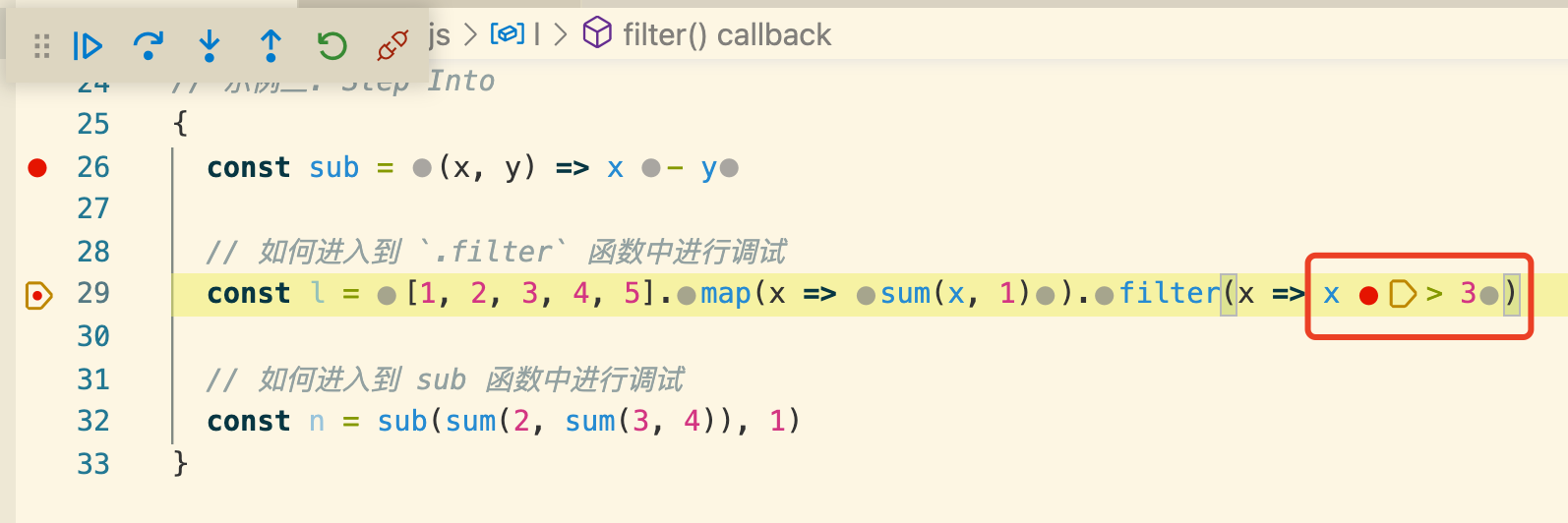### # 在单行调用多个函数表达式时，如何设置条件断点

``````// 如何在 map 函数中，当 x === 3 时打断点
const l = [1, 2, 3, 4, 5].map(x => sum(x, 1))
``````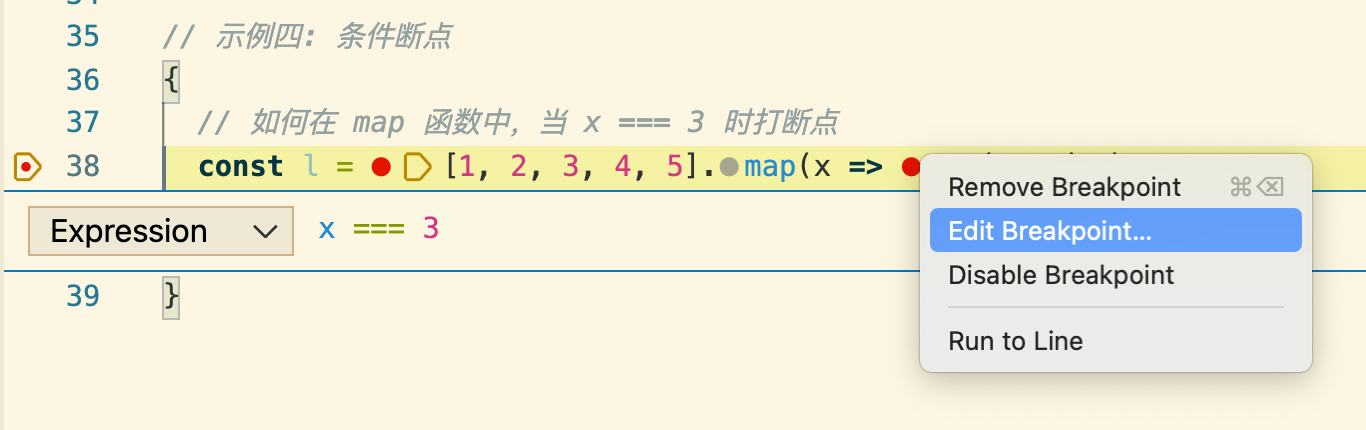## # Promise/Async

`Node` 与浏览器环境中，关于 `promise` 的调试有所不同，由于 `async_hooks` 的存在，node 中的调试经常会陷入无关的系统源码，而在浏览器环境中就简单很多。

### # 如何进入到 `promise.then` 函数中进行调试？

``````Promise.resolve(3).then(o => {
// 如何 StepOver/StepInto 到当前行进行调试
console.log(o)
})

console.log('hello, world')
``````

### # 如何跳进 `await` 的函数中进行调试？

``````const sleep = (seconds) => {
// 从 await sleep(2000) 如何调试到这里边
console.log('DEBUG TO HERE')
return new Promise(resolve => setTimeout(resolve, seconds))
}

await sleep(2000)
``````

### # 以下 `sum` 函数和 `asyncSum` 函数 `Step Into` 的步骤是否一致？

``````function sum (a, b) {
return a + b
}

async function asyncSum (a, b) {
return a + b
}

async function main () {
const r1 = await sum(3, 4)
const r2 = await asyncSum(3, 4)
}
``````

## # Error

### # 当发生异常时，如何直接断点到异常位置调试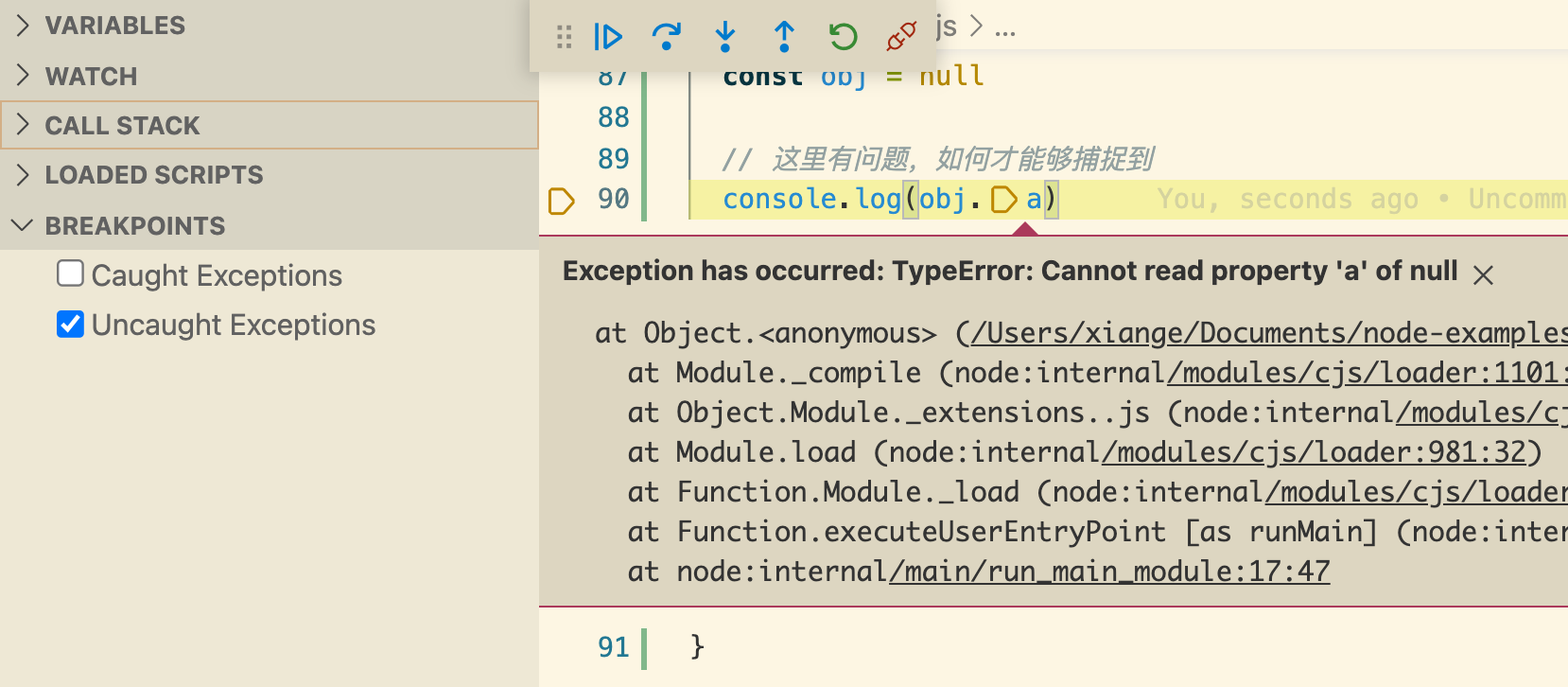## # 总结

1. 在调试源码(React/Vue)时有哪些技巧？
2. 如何在 Node/VSCode 中调试？
3. 如何更好地调试 Node/C++ 跨语言代码？

``````// 示例一: Return Value
{
const expansiveCompute = (a, b) => a + b

function sum(a, b) {
// 在调试中如何得到 7 这个结果
return expansiveCompute(a, b)
}

sum(3, 4)
}

// 示例二: Step Over
{
const sum = (a, b) => a + b

// 当在改行设有断点时，Step Over 的下一步是跳过一行还是表达式？
const l = [1, 2, 3, 4, 5].map(x => sum(x, 1)).filter(x => x > 3)

// 此行同理
const n = sum(sum(3, 4), 1)
}

// 示例三: Step Into
{
const sub = (x, y) => x - y

// 如何进入到 `.filter` 函数中进行调试
const l = [1, 2, 3, 4, 5].map(x => sum(x, 1)).filter(x => x > 3)

// 如何进入到 sub 函数中进行调试
const n = sub(sum(2, sum(3, 4)), 1)
}

// 示例四: 条件断点
{
// 如何在 map 函数中，当 x === 3 时打断点
const l = [1, 2, 3, 4, 5].map(x => sum(x, 1))
}

// 示例五: Promise
{
Promise.resolve(3).then(o => {
// 如何 StepOver/StepInto 到当前行进行调试
console.log(o)
})

console.log('hello, world')
}

// 示例六: Promise
{
async function main() {
const sleep = (seconds) => {
// 从 await sleep(2000) 如何调试到这里边
console.log('DEBUG TO HERE')
return new Promise(resolve => setTimeout(resolve, seconds))
}

await sleep(2000)
}

main()
}

// 示例七: async/await
{
function sum (a, b) {
return a + b
}

async function asyncSum (a, b) {
return a + b
}

async function main () {
// 以下两行断点调试有何不同
const r1 = await sum(3, 4)
const r2 = await asyncSum(3, 4)
}

main()
}

// 示例八: 如何快速发现有问题的代码
{
const obj = null

// 这里有问题，如何才能够捕捉到
console.log(obj.a)
}
``````

## 关于山月

Last Updated: 7/21/2019, 11:25:08 AM